The Polyhedral Angles ClipArt gallery offers 13 images of angles formed by the intersection of three or more planes at a common point. Included are illustrations of dihedral and trihedral angles.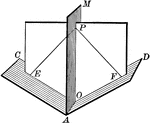### Dihedral Angle With Bisector

Illustration of a dihedral angle bisected by a plane. "Every point in a plane which bisects a dihedral…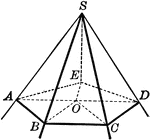### Convex Polyhedral Angle

Illustration of a convex polyhedral angle. "The sum of the face angles of any convex polyhedral angle…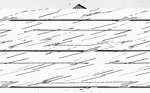### Dihedral Angle

Illustration of dihedral angle AB (or M-AB-N) formed by planes.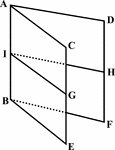### Dihedral Angle

Illustration of dihedral angle AB formed by planes.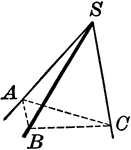### Polyhedral Angle

Illustration of polyhedral angle. "The opening of three or more planes which meet at a common point…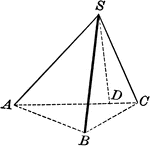### Trihedral Angle

Trihedral angle. "The sum of any two face angles of a trihedral angle is greater than the third face…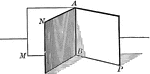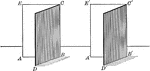### Two Equal Dihedral Angles

Illustration of 2 equal dihedral angles.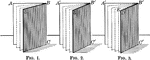### Equal Dihedral Angles

Illustration of 3 equal dihedral angles. "Two dihedral angles have the same ratio as their plane angles."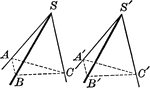### Equal Polyhedral Angles

Illustration of two polyhedral angles that are equal.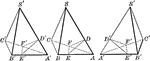### Equal trihedral angles

Illustration used to show that two trihedral angles are equal or symmetrical when the three face angles…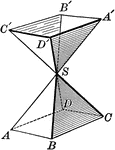### Symmetrical Polyhedral Angles

Illustration of two symmetrical polyhedral angles.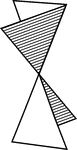### Vertical Dihedral Angles

Illustration of two vertical dihedral angles.